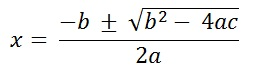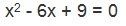Study Materials: CBSE Notes

Our CBSE Notes for introduction-Natures of root Class 10 maths 4. Quadratic Equations - CBSE Notes - Toppers Study is the best material for English Medium students cbse board and other state boards students.

Notes ⇒ Class 10th ⇒ Mathematics ⇒ 4. Quadratic Equations

# introduction-Natures of root Class 10 maths 4. Quadratic Equations - CBSE Notes - Toppers Study

Topper Study classes prepares CBSE Notes on practical base problems and comes out with the best result that helps the students and teachers as well as tutors and so many ecademic coaching classes that they need in practical life. Our CBSE Notes for introduction-Natures of root Class 10 maths 4. Quadratic Equations - CBSE Notes - Toppers Study is the best material for English Medium students cbse board and other state boards students.

## introduction-Natures of root Class 10 maths 4. Quadratic Equations - CBSE Notes - Toppers Study

CBSE board students who preparing for class 10 ncert solutions maths and Mathematics solved exercise chapter 4. Quadratic Equations available and this helps in upcoming exams 2023-2024.

### You can Find Mathematics solution Class 10 Chapter 4. Quadratic Equations

• All Chapter review quick revision notes for chapter 4. Quadratic Equations Class 10
• NCERT Solutions And Textual questions Answers Class 10 Mathematics
• Extra NCERT Book questions Answers Class 10 Mathematics
• Importatnt key points with additional Assignment and questions bank solved.

NCERT Solutions do not only help you to cover your syllabus but also will give to textual support in exams 2023-2024 to complete exercise - maths class 10 chapter 4 in english medium. So revise and practice these all cbse study materials like class 10 maths chapter - in english ncert book. Also ensure to repractice all syllabus within time or before board exams for ncert class 10 maths ex - in english.

See all solutions for class 10 maths chapter 4 exercise 4 in english medium solved questions with answers.

### introduction-Natures of root class 10 Mathematics Chapter 4. Quadratic Equations

• Introduction-Natures Of Root Class 10 Maths 4. Quadratic Equations - CBSE Notes - Toppers Study
• Class 10 Ncert Solutions
• Solution Chapter 4. Quadratic Equations Class 10
• Solutions Class 10
• Chapter 4. Quadratic Equations Introduction-Natures Of Root Class 10

## introduction-Natures of root Class 10 maths 4. Quadratic Equations - CBSE Notes - Toppers Study

Introduction:

The equation ax2 + bx + c = 0, is the standard form of a quadratic equation, where a, b and c are real numbers and a ≠ 0.

Example:

1. 3x- 5x = 0,

This equation can be expressed in the form of ax2 + bx + c = 0. then

a = 3, b = -5, c = 0,

Here c = 0, As Term c is disappear.

This also showing a ≠  0.  Hence this is a quadratic equation.

2. 5x2 + 2x -7=0,

Here a = 5, b = 2, c = -7, so it can be also expressed in the form of ax2 + bx + c =0,

3. 3x2 ,

This is single term polynomial i.e mononomial. It can be also expressed in the form of ax2 + bx + c = 0. In which a= 3, b = 0, c = 0,  Here b = 0, c = 0 but there is no a ≠  0.

So, this is also a quadratic equation.

4. 4x + 9,

This cannot be expressed in the form of ax2 + bx + c = 0. As the ax2 term is disappear. Hence a = 0. Which can not fulfill the condition of to be a quadratic equation.

• All quadratic polinomials can be expressed in the form of quadratic equation ax2 + bx + c = 0.
• ax2 + bx + c = 0, a ≠ 0 is called the standard form of a quadratic equation.

Another equations which are not a quadratic equation.

1. x3 + 3x2 + 4x + 5, 2x3 + 4x, 4x3 - 5x2 + 7 and all cubic polynomials.

2. All linear equations like 4x + 3, 5x, 7x + 2 etc.

4. Polynomials of power more than 2 and less than 2.Nature of Roots:

• Each quadratic equation has two roots. they are said to be α and β
• A real number α is said to be a root of the quadratic equation ax2 + bx + c = 0, a ≠ 0. If ax2 + bx + c = 0, the zeroes of quadratic polynomial ax+ bx + c and the roots of the quadratic equation ax2 + bx + c = 0 are the same.
• The roots of a quadratic equation ax2 + bx + c = 0, a  ≠  0 gives;Where  b2 - 4ac ≥ 0.

Since b2 – 4ac determines whether the quadratic equation ax2 + bx + c = 0 has real roots or not, b2 – 4ac is called the discriminant of this quadratic equation and Discriminant is denoded by capital Letter D

Hence,

D = b2 – 4ac,

Nature of Roots of Quadratic Equations:

Nature of Roots:See here b2 - 4ac given in under root.

This valuue b2 - 4ac is called Discriminant.

Which is denoted by "D".

∴ D = b2 - 4ac

[ Nature of root is determined by the value of Discriminant;]

There are three natures of roots.

(a)  D = 0; [Two equal and real roots, if b2 - 4ac = 0 or (D = 0)]

Example:Solution:

x2 - 6x + 9 = 0

a = 1, b = -6, c = 9

Checking for existance of roots,

D = b2 - 4ac

D = (-6)2 - 4 × 1 × 9

D = 36 - 36

D = 0

Hence D = 0

∴ There is two equal and real roots [Nature-I ]

This equation gives two equal and real roots x = 3, and x = 3.

Such equation which have equal and real root is also called a complete square equation.

(b) D > 0; [ Two real and distinct root]

Example;

7x2 + 2x - 3 = 0

Solution:

7x2 + 2x - 3 = 0

a = 7, b = 2, c = -3

Checking for existance of roots,

D = b2 - 4ac

D = (2)2 - 4 × 7 × -3

D = 4 - (-84)

D = 4 + 84

D = 88

Hence D > 0

∴ There is two real and distinct roots [Nature-II]

(c) D < 0; No Real roots

Example

8x2 + 5x + 3 = 0

Solution:

8x2 + 5x + 3 = 0

a = 8, b = 5, c = 3

Checking for existance of roots,

D = b2 - 4ac

D = (5)2 - 4 × 8 × 3

D = 25 - 96

D = -71

Hence D < 0

∴ There is no roots [Nature-III]

##### Other Pages of this Chapter: 4. Quadratic Equations

Important Study materials for classes 06, 07, 08,09,10, 11 and 12. Like CBSE Notes, Notes for Science, Notes for maths, Notes for Social Science, Notes for Accountancy, Notes for Economics, Notes for political Science, Noes for History, Notes For Bussiness Study, Physical Educations, Sample Papers, Test Papers, Mock Test Papers, Support Materials and Books.Mathematics Class - 11th

NCERT Maths book for CBSE Students.

books

## Study Materials List:

##### Notes ⇒ Class 10th ⇒ Mathematics
1. Real Numbers
2. Polynomials
3. Pair of Linear Equations in Two Variables
5. Arithmetic Progressions
6. Triangles
7. Coordinate Geometry
8. Introduction to Trigonometry
9. Some Applications of Trigonometry
10. Circles
11. Constructions
12. Areas Related to Circles
13. Surface Areas and Volumes
14. Statistics
15. Probability

New Books